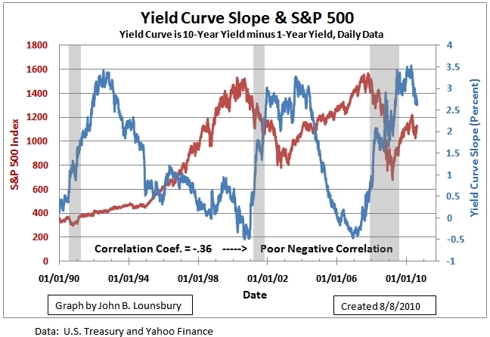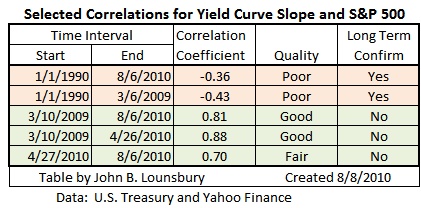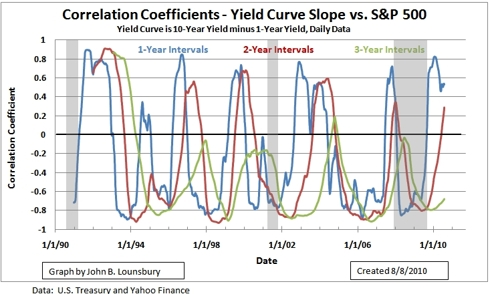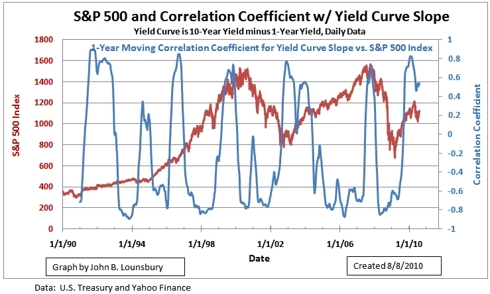Please Note: Blog posts are not selected, edited or screened by Seeking Alpha editors.

What Does the Slope of the Yield Curve Predict for Stocks?

|Includes:

In a previous article, which presented the analysis of reader Albertarocks, an interesting relationship was discussed between the ten-year Treasury yield and movement in the stock market. This gave rise in this analyst's mind to thoughts about the slope of the yield curve.

That led to the exercise summarized here to look for a relationship between the slope of the yield curve and the performance of stocks.  These are compared in the next graph.Over the past 20 years there is a poor negative correlation between the slope of the yield curve and the value of the S&P 500.  When we look at shorter time intervals the correlation can be much different at various times.  Some examples are shown in the following table.The above table raises the question of just how correlations might change for various correlation periods over a longer time period.  The following graph plots 1-, 2- and 3-year moving correlations over the time period 1990 to 2010.The correlation coefficient value varies in a cyclic fashion for  short-term correlations of 1-, 2- and 3-years.  It appears that, as the interval of correlation increases the amplitude of the oscillation decreases.  For the longer interval of three years, most of the 20-year time period shows negative correlation.  For 1- and 2-year intervals the correlation repeatedly cycles into positive territory.

It does not appear that deviations in correlations for short intervals of one or two years from longer term correlations can be used to predict what can be expected from the stock market.  This is a topic that deserves more in-depth research.

The preliminary work does not indicate that the slope of the yield curve gives much if a clue in predicting stock market movement.  For example, see the following graph where the S&P 500 index has been overlaid the one year moving correlation coefficient for the yield curve slope with the S&P 500 index (blue line in the above graph and below).The title question:  What does the slope of the yield curve predict for stocks?
The answer after this brief exercise:  Apparently not much.

Disclosure: Long several S&P 500 stocks. Long and short posiitions in some Nasdaq stocks.